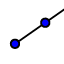# Sum of Exterior Angles of a Triangle

Topic:
Angles

## Constructing the Exterior Angles of a triangle

1. On the open Geogebra window below, use the segment toolto construct a non-regular triangle. 2. Label the vertices A, B and C using the text tool.3. Construct one set of exterior angles using the ray tool. Make sure your exterior angles all go the same way, like the picture below. Label the exterior parts of the angles D (next to A), E (next to B) and F (by C).

## Construct the triangle and exterior angles below.

4. Measure angles DAB, EBC and FCA. Select either the two lines that make the angle or 3 vertices to measure the angle. Make sure to measure the exterior angles less than 180.

What is the sum of the 3 exterior angles?

## 6. Drag your vertices around and allow the angles to change. Add them again.

What is the sum of the exterior angles now?

Complete the following conjecture: The sum of the exterior angles of a triangle is ___________